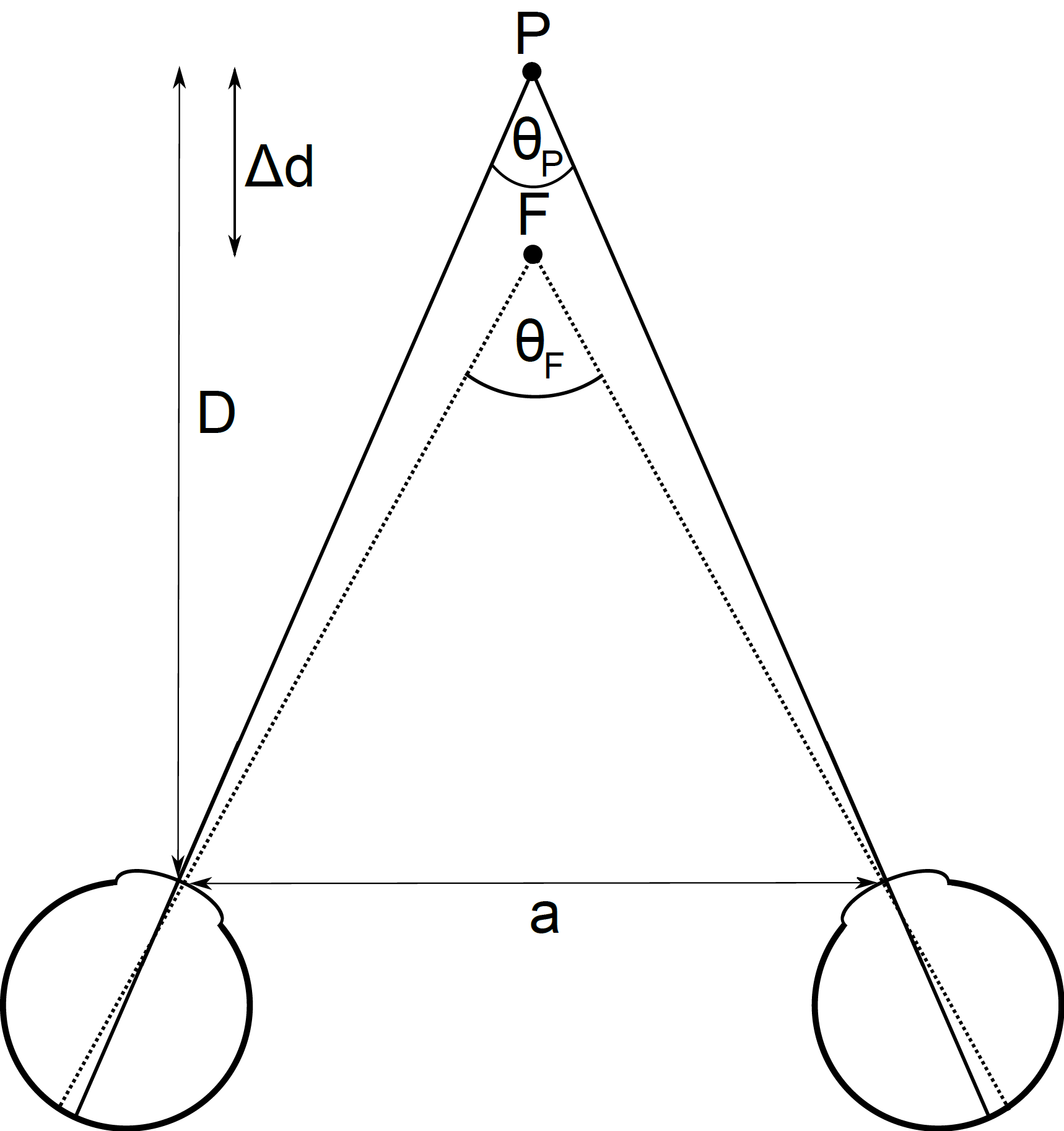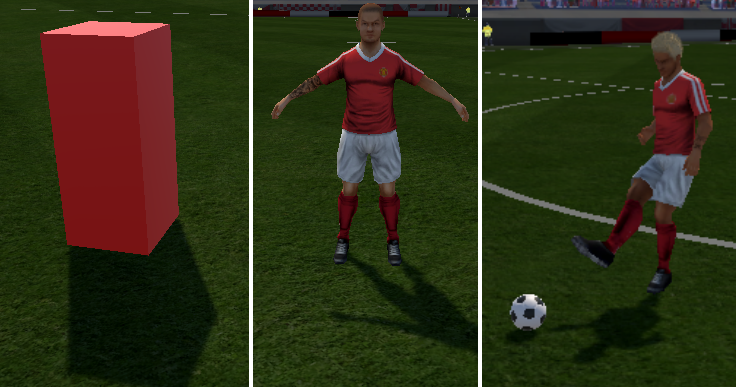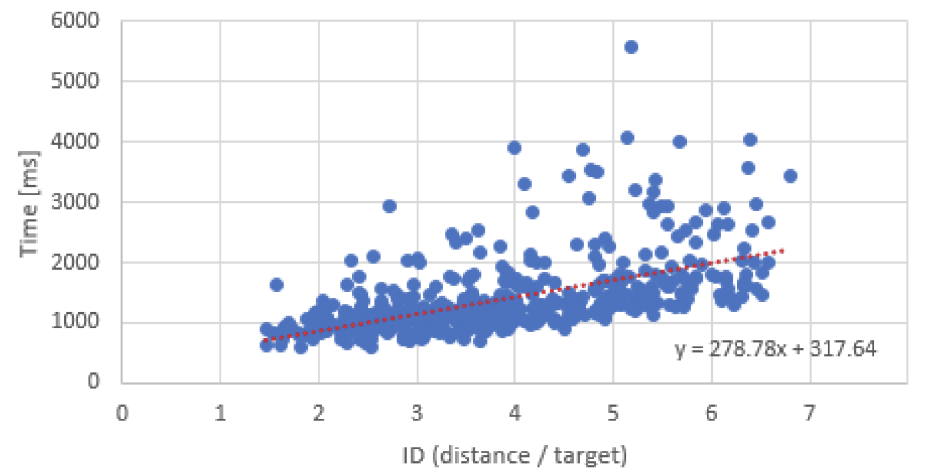# Human-Computer Interaction (HCI)

Novel interaction methods and models for wearable and ubiquitous systems are explored. The aim is to provide intuitive and innovative user interfaces for being able to assess user behavior. Our research methods focus on computer graphics in the context of HCI, the effect of extended reality fidelity and consequently different performance measures along the reality-virtuality continuum to validate such environments.

### Computer Graphics in the Context of HCI

In order to assess the binocular functions of humans (i.e. stereopsis or depth perception) and to monitor the performance increase after training, it is important to have standardized levels of difficulty. Usually, stereopsis performance is evaluated as the minimal distance ($\Delta d$) between objects ($P$ and $F$) still perceivable by the observer at a base distance ($D$) and an individual interpupillary distance ($a$). Given the distances between observer and objects ($\Delta d$ and $D$) and between the eyes of the observer ($a$), the minimal distance can be described as the difference of the angles at the objects $P$ and $F$ spanning the eyes of the observer ($\theta_F$ and $\theta_P$). This is called the disparity difference $\Theta = \theta_F - \theta_P$ and is usually given in minutes or seconds of arc.If we seek a constant $\Theta$ and we set or know the base distance $D$ we can calculate the distance $d_F = D - \Delta d$.

\begin{aligned} & \Theta = \theta_F - \theta_P \\ \\ & \Theta + \theta_P = \theta_F\\ \\ \\ & \theta_F = 2 * tan^{-1}(\frac{a}{2d_F})\\ \\ \\ & \Theta + \theta_P = 2 * tan^{-1}(\frac{a}{2d_F})\\ \\ & \frac{\Theta + \theta_P}{2} = tan^{-1}(\frac{a}{2d_F})\\ \\ & tan(\frac{\Theta + \theta_P}{2}) = \frac{a}{2d_F}\\ \\ & \frac{a}{2*tan(\frac{\Theta + \theta_P}{2})} = d_F\\ \\ \end{aligned}

This is the case in which the two objects have the same horizontal distance to the eyes. If there is any offset on the horizontal axis, the offset has to be incorporated and a quadratic system of equations is yielded.

### User Interface FidelityFidelity in terms of HCI can be seen as the degree to which a prototype or interface recreates a real-world experience. This, on the one hand, incorporates the simulation detail of the created interface (e.g. how realistic is the look and behavior of a human avatar presented to the user in virtual reality). On the other hand, the degree of interaction can be applied as a method to explore the efficiency of a user interface. Especially for extended realities, this plays a key role since they provide new interaction (e.g. free hand-interaction in a 3D space) and visualization possibilities. Therefore the effect of different fidelity levels on user performance for specific tasks is investigated in our research. One example in team sports would be the influence on anticipation and decision-making of athletes when experiencing virtual teammates varying in kinematic and kinetic fidelity.

### Performance MeasuresTo evaluate the feasibility and efficiency of user interfaces for a diversity of tasks, subjective and objective performance measures are used in our research. Therefore subjective measures describe a user’s self-introspection with respect to a specific action. This can be done pre-, peri- or post-experimental by using different methods like questionnaires, cognitive walkthrough or think aloud. Contrary, objective measures enable the evaluation of a user’s performance based on automated computational methods and hence are free of human-bound influences like self-esteem, emotional attachment, and sympathy. Objective measures are heavily dependent on the underlying task or use case to be evaluated. Some broadly used metrics are task completion time or the activity level of the user.